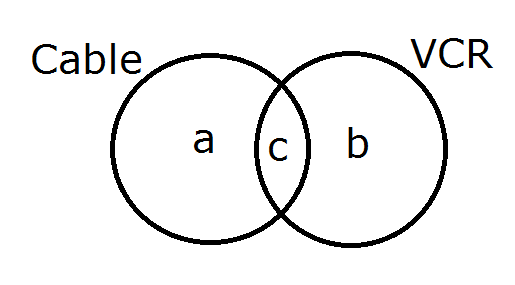Question 26

# In a locality, two-thirds of the people have cable TV, one-fifth have VCR, and one-tenth have both. What is the fraction of people having atleast one among cable -TV and VCR?

SolutionLet the distribution of people having cable TV and VCR be as given in the diagram above.
Hence, $$a+c =\frac{2}{3}$$
$$b+c=\frac{1}{5}$$
and $$c=\frac{1}{10}$$

We need to find $$a+b+c=?$$
This equals $$(a+b)+(b+c)-c = \frac{2}{3}+\frac{1}{5}-\frac{1}{10}$$
Which equals $$\frac{20+6-3}{30}=\frac{23}{30}$$